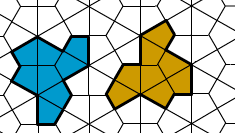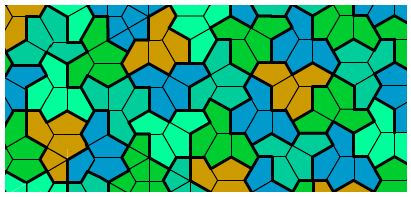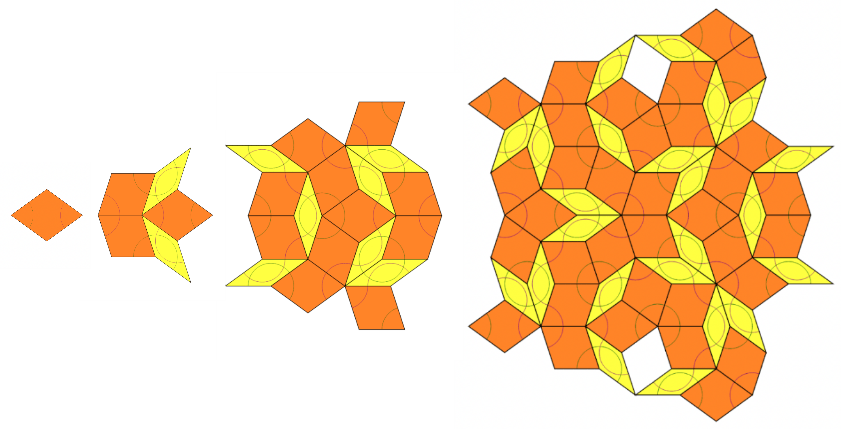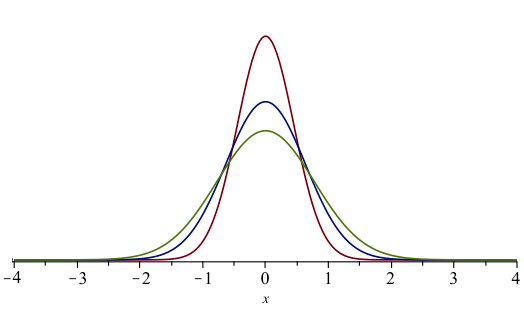# Tony’s Take March 2023

## This month’s topics:

### Aperiodic tilings in The New York Times

Siobhan Roberts contributed “Elusive ‘Einstein’ Solves a Longstanding Math Problem” to the Times on March 28, 2023. The longstanding problem is the existence of a 2-dimensional shape that can tile the plane but only aperiodically: the pattern never repeats no matter how far out you go. Roberts starts by summarizing its history, which goes back some sixty years and has intriguing connections with “undecidability” problems in logic. There was an explosion of interest in the problem around the time of the discovery of the aperiodic Penrose tilings (they made the cover of Scientific American in January, 1977). But the simplest Penrose tiling uses two different tiles, and the question still remained if the phenomenon could be achieved with just one.

The breakthrough was announced in the ArXiV posting An aperiodic monotile (the title says it all) on March 20. Among the authors, David Smith, Joseph Samuel Myers, Craig S. Kaplan and Chaim Goodman-Strauss, only Kaplan (University of Waterloo) and Goodman-Strauss (University of Arkansas, Fayetteville) are academics. The tile — known as “the hat” for its fedora-ish shape — was discovered by Smith. But the proof that it, and its mirror image, could tile the plane, and could only do it aperiodically, is extremely intricate and took the whole team.The “hat” tile and its mirror image. Note that the tile is a union of elements of a regular hexagonal-symmetry tiling of the plane.Part of a tiling of the plane using the hat (shades of green and blue) and its mirror image (yellow). There are uncountably many different such tilings.

Roberts goes much deeper than usual into the nitty-gritty details of the work. For example, the Times displays this illustration of the intricate recursive nature of the tilings, the key to generating larger and larger assemblies of the tiles and to proving that they can cover the whole plane.The first four subdivision/inflation steps of a procedure leading to an aperiodic monotiling of the entire plane. Image courtesy of Craig Kaplan.

These images can be compared with the corresponding ones for a Penrose tiling. The gnarly geometry of the hat tile makes the procedure here considerably more complicated.The first four subdivision/inflation steps for a Penrose tiling of the plane. Images by Taktaal, used under the CC BY-SA 4.0 license

### The New York Times covers the Abel Prize

More from the Gray Lady. On March 22, this year’s Abel Prize was awarded to Luis Caffarelli of the University of Texas, Austin. Kenneth Chang covered the award for the Times, calling it “like a Nobel Prize for mathematics”. The print-edition headline was “Math Whiz Awarded Abel Prize for Applying Equations to Real-Life Problems.”

The Abel Prize site cites Caffarelli’s “seminal contributions to regularity theory for nonlinear partial differential equations.” Real-life problems often involve partial differential equations. A simple and important example is the 1-dimensional heat equation $$\frac{\partial u}{\partial t} = k\frac{\partial^2 u}{\partial t^2}$$ governing the flow of heat in an insulated bar. In the heat equation, $u$ is temperature; at any point, the time-derivative of $u$ is proportional ($k$ is a positive constant depending on what the bar is made of) to its second space-derivative. In general, a partial differential equation describes how the change of a measured quantity (in this case, temperature) with respect to one variable (often time) is related to the way it changes with respect to another variable (in this case, space).Solutions to the heat equation: temperature distribution in an insulated bar at three equally spaced points in time $t_1$ (red) $< t_2$ (blue) $< t_3$ (green). Notice how the change in temperature is proportional to the convexity of the graph.

The equations Caffarelli studies are more complicated (note the word non-linear in the citation). Chang gives three examples of where such equations occur in real-life phenomena.

Melting ice: How does the shape of an ice-cube, for example, change as it melts? If the ice-cube is not a perfect sphere, some parts will melt more quickly than others, so the problem itself changes with time.

The obstacle problem: In Chang’s example, a balloon is pressed against a wall. What shape does it take? If the wall is flat, we can imagine what the solution would look like, but what if the wall has a protrusion? The shape of the balloon changes as you move both side-to-side and up-and-down along the wall. These two directions correspond to two variables in the equation.

The Navier-Stokes equation: This is the target of one of the Millenium Prizes. It describes the flow of a fluid like water or air. Obviously very important, but we still cannot prove that solutions to the equation are reasonably behaved. For example, we don’t know whether the speed will always stay finite if it starts out with certain properties that you would expect from a real-life fluid. Chang tells us that Caffarelli, along with the late Louis Nirenberg and Robert Kohn (both then at the Courant Institute, NYU), was able to show that “regions of infinite speed, if they existed, would have to be extremely small.”

### The geometry of color space

Our perception of color is mediated by the cone cells in our retina. These come in three types, each one “tuned” (responding maximally) to a certain wavelength: red, green or blue. So our color space is naturally 3-dimensional. But what about its geometry? Can we quantify our feeling that, say, blue is closer to purple than to yellow? In 1855 Hermann von Helmholz published his experiments showing that the Euclidean distance in the (red, green, blue) coordinate system does not correspond to the way we perceive differences between colors (see a recent paper by Giulio Peruzzi and Valentina Roberti).

These ideas developed over the next fifty years. Researchers viewed color space as an example of a Riemannian manifold, a space where the distance between two points can be defined as the length of the geodesic (the shortest curve) between them. If we use the Euclidean distance, a geodesic is a straight line, but this is not true in every Riemannian space. (Riemann himself had given the space of colors as an example of a manifold that everyone should be familiar with).

It turns out that this concept only goes part way in modeling perceptual reality. “Scientists disprove 100-year-old understanding of color perception” was posted on Newswise March 16, 2023. The item is a press release from Los Alamos National Laboratory calling attention to a paper titled “The non-Riemannian nature of perceptual color space”, published in PNAS on April 29, 2022. The authors are five members of their research staff, a team led by Roxana Bujack and Terece Turton. They show that the way distances work in color space is incompatible with the geodesic definition of differences. This is because of the perceptual phenomenon known as diminishing returns. A 2018 conference paper written by Bujack and others describes diminishing returns this way: “When presented with two colors, $A$, $C$, and their perceived middle (average/mixture) $B$, an observer usually judges the sum of the perceived differences of each half greater than the difference of the two outer colors $\Delta(A, B) + \Delta(B, C) > \Delta(A, C)$.” In a Riemannian manifold, the average $B$ would be halfway along the geodesic from $A$ to $C$, and this equation would read $\Delta(A, B) + \Delta(B, C) = \Delta(A, C)$.Here three colors $A, B, C$ are situated on a geodesic in color space. As the reported experiments show, $\Delta(A,C)$ is strictly smaller than the sum of the others, differing from what you would see in a Riemannian manifold.

The authors refer to the Weber–Fechner law: the intensity of a sensation is proportional to the logarithm of the intensity of the stimulus causing it (as reported in Merriam-Webster Medical, where it is characterized as “an approximately accurate generalization in psychology.”) This law is often taken for granted. For example, we measure loudness in decibels, which are logarithmic units: a sound 10 decibels louder than another has 10 times the physical intensity. The authors suggest that the non-Riemannian phenomena they are detecting may correspond to a “second-order Weber–Fechner law describing perceived differences.”